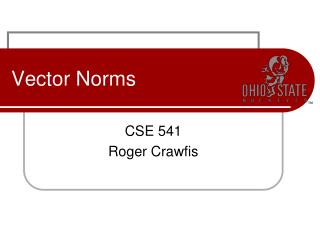DownloadDownload PresentationVector Norms

# Vector Norms

Télécharger la présentation## Vector Norms

- - - - - - - - - - - - - - - - - - - - - - - - - - - E N D - - - - - - - - - - - - - - - - - - - - - - - - - - -
##### Presentation Transcript

1. Vector Norms CSE 541 Roger Crawfis

2. Vector Norms • Measure the magnitude of a vector • Is the error in x small or large? • General class of p-norms: • 1-norm: • 2-norm: • -norm:

3. Properties of Vector Norms • For any vector norm: • These properties define a vector norm

4. Matrix Norms • We will only use matrix norms “induced” by vector norms: • 1-norm: • -norm:

5. Properties of Matrix Norms • These induced matrix norms satisfy:

6. Condition Number • If A is square and nonsingular, then • If A is singular, then cond(A) =  • If A is nearly singular, then cond(A) is large. • The condition number measures the ratio of maximum stretch to maximum shrinkage:

7. Properties of Condition Number • For any matrix A, cond(A)  1 • For the identity matrix, cond(I) = 1 • For any permutation matrix, cond(P) = 1 • For any scalar , cond(A) = cond(A) • For any diagonal matrix D,

8. Errors and Residuals • Residual for an approximate solution y to Ax = b is defined as r = b – Ay • If A is nonsingular, then ||x– y|| = 0 if and only if ||r || = 0. • Does not imply that if ||r||<e, then ||x-y|| is small.

9. Estimating Accuracy • Let x be the solution to Ax = b • Let y be the solution to Ay = c • Then a simple analysis shows that • Errors in the data (b) are magnified by cond(A) • Likewise for errors in A